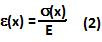## Wednesday, April 8, 2009

### Irodov Problem 1.298Consider a section of the rod XB that is x units long from the lowest end of the rod. Let the density of the rod be p and its cross-section area be S. There are two forces acting on this part of the rod, i) the force of gravity pxSg pulling it down and ii) the force due to stressin the rod at point X given by. Since the section of the rod is not falling down we have,The strain experienced by the section XB of the rod is thus given by,Suppose that the change in length for the section XB of the rod is, then we have,The net strain in the rod is thus given by,(b) In addition to the longitudinal strain that elongates the rod, the rod also experiences a lateral strain (dictated by Poisson's ratio) that leads to a reduction in the rod's radius given by. In other words if the radius of the rod is R, then the change in the radius is given by,The change in volume of the rod is given by,